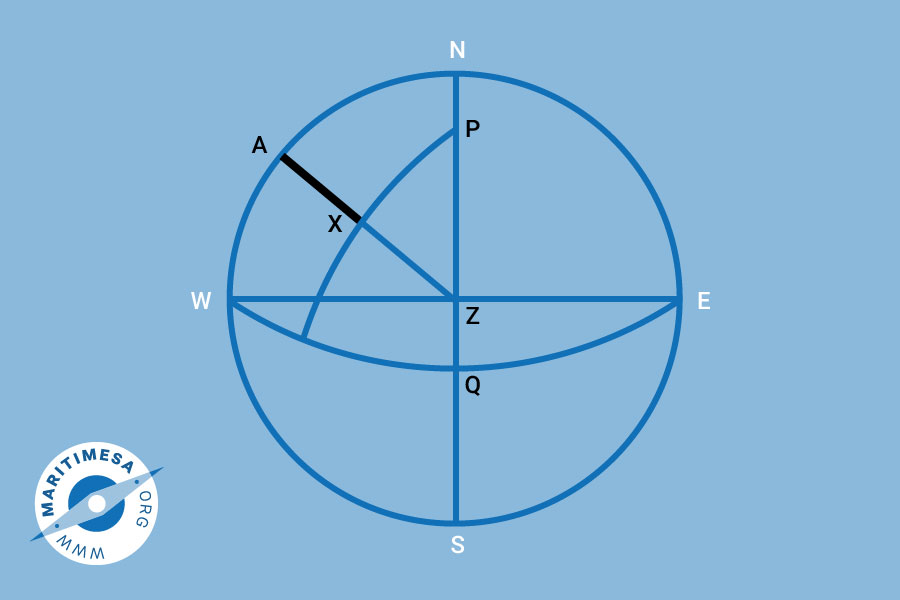# The spherical triangle

During the discussion of the celestial sphere last year, we discussed the spherical triangle PZX which was formed by the projected positions of the celestial pole (P), the observer’s zenith (Z) and the celestial body (X), on the celestial sphere. It was stated that the solution of this triangle formed the basis of all astronomical navigation computations.The spherical triangle.

The three sides of the triangle are the following:

• PZ = This is the arc of the great circle that joins the elevated pole (P) and the observer’s zenith and forms part of the observer’s meridian. The angular distance from the observer’s zenith to the celestial pole is the complement of the observer’s latitude. PZ (when used) is referred to as the “co-lat”.
• PX = The arc of a great circle which joins the pole and the celestial body (X) is part of the celestial meridian that passes through the body. The angular distance of the body from the pole is the complement of the declination and is referred to as the “co-dec) (when used). It is also referred to as the polar distance. If the name of the declination is opposite to that of the latitude, then the length of the side PX must be greater than 90°. If this is the case, then PX is equal to 90° + declination.
• ZX = The arc of the great circle that joins the zenith and the body is part of the vertical circle which passes through the body. Since the altitude of the zenith is 90° then ZX or zenith distance (if used) is found by taking the complement of the altitude of the body.

The three angles of the triangle are:

• P = The angle at the pole between the observer’s meridian and the meridian passing through the body. This is similar to the definition of the LHA (local hour angle). The angle P is always considered to be less than 180°. When the body is to the west of the observer’s meridian (the body is setting), it will be the LHA of the body. If the body is to the east of the observer’s meridian (body rising), it will be 360° – LHA. Angle P is found from the GHA of the body and the observer’s longitude.
• Z = The angle between the observer’s meridian and the vertical circle which passes through the body. This is the azimuth which is measured from 0 to 180° east or west of the elevated pole. From the azimuth, the bearing of the celestial body is obtained.
• X – This is not used.